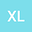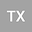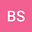Non-singular calculation of geomagnetic vectors and geomagnetic gradient tensors
•••• +3Bijiao Sun
Xi'an Research Institute of Surveying and Mapping
Author ProfileXinxing Li
Information and Engineering University
Author ProfileYunpeng Wang
Xi'an Research Institute of Surveying and Mapping
Author ProfileBin Guan
Xi'an Research Institute of Surveying and Mapping
Author Profile## Abstract

Some spherical harmonic expressions (SHEs) of gravitational and geomagnetic field elements will become infinite when computation point approaches polar regions, as the sine function of the geocentric co-latitude contained in the denominator tends to be zero. Currently, this singularity problem has been solved for gravitational field case, however, it remains unsolved for geomagnetic vectors (GVs) and geomagnetic gradient tensors (GGTs). The reason is that the latter use Schmidt semi-normalized associated Legendre function (SNALF), which is different from fully-normalized associated Legendre function (FNALF) used in the former. To overcome this singularity problem, we derive new non-singular expressions of the first- and second-order derivatives of Schmidt SNALF, and the corresponding two kinds of spherical harmonic polynomials. When the novel expressions are applied to the traditional formulae of GVs and GGTs, more practical expressions of GVs and GGTs with non-singularity are formulated by refining the cases that the order m equals 0, 1, 2 and other values. Furthermore, to provide flexible calculation strategies for Schmidt SNALF, we derive four kinds of recursive formulae, including the standard forward row recursion (SFRR), the standard forward column recursion (SFCR), the cross degree and order recursion (CDOR), and the Belikov recursion (BR). Besides, we demonstrate the effectiveness of the new derived non-singular expressions of GVs and GGTs and analyze the computation speed and stability of the four recursive formulae of Schmidt SNALF by extensive numerical experiments. Results achieve significant improvements in solving the singularity problem of the SHEs of GVs and GGTs.
Dec 2021Published in Journal of Geophysical Research: Solid Earth volume 126 issue 12. 10.1029/2021JB023397